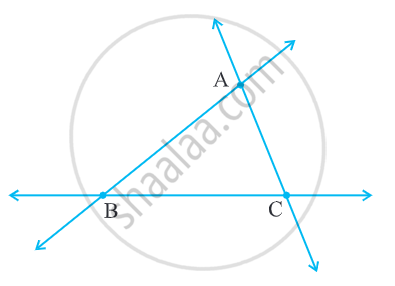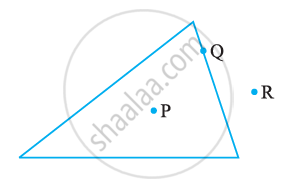# Concept of Triangles - Sides, Angles, Vertices, Interior and Exterior of Triangle

#### definition

Triangle: A triangle is a closed figure made by joining three non-collinear points by line segments. A triangle is a three-sided polygon.

# Triangles:• A triangle is a three-sided polygon. In fact, it is the polygon with the least number of sides.

• A triangle is a closed figure made by joining three non-collinear points by line segments.
• It has three sides, three angles, and three vertices.

• The vertices, sides and angles of a triangle are called the parts of the triangle.

• We write ∆ABC instead of writing "Triangle ABC".

• ‘Length of line segment AB’ is written as l(AB).

• The three sides of the triangle are bar"AB", bar"BC", and bar"CA".
The three angles are ∠BAC, ∠BCA, and ∠ABC.
The points A, B, and C are called the vertices of the triangle.• Being a polygon, a triangle has an exterior and an interior.

• P is in the interior of the triangle, R is in the exterior and Q on the triangle.
If you would like to contribute notes or other learning material, please submit them using the button below.

### Shaalaa.com

Triangle [00:10:13]
S
0%